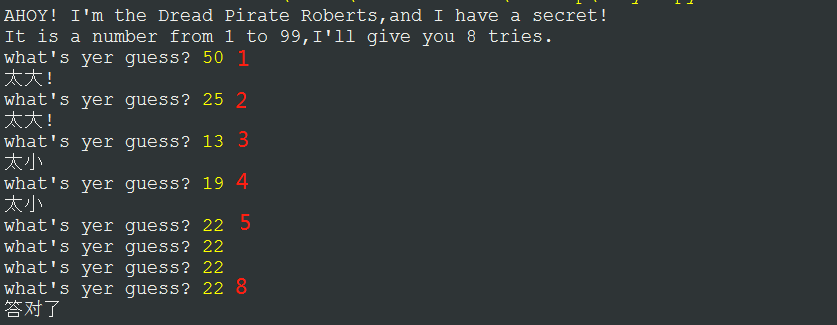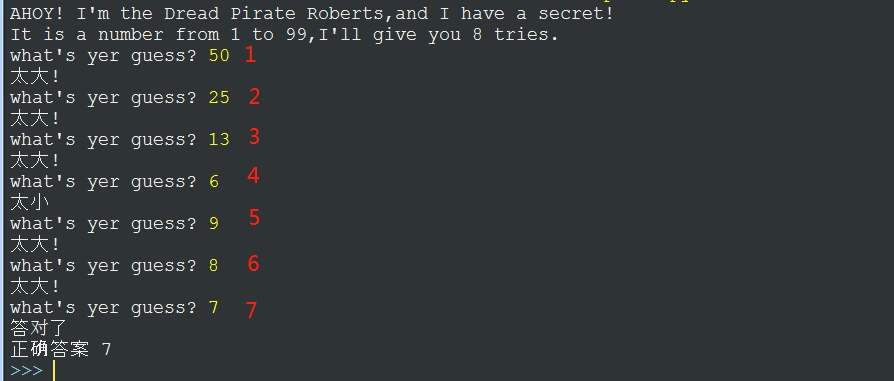1.Python中我按照python 2的教材在python 3 上写了一个猜数小游戏 但是运行之后 我写的代码在我猜对了数之后不会跳出循环, 而是又一次的开始了循环，直到次数用完。后来找大神改了一下代码可以成功运行了，但是对于其中 guess=int（input（""））和 if int（guess）有了疑问。

2.教材上的代码：

``````import random
secret = random.randint(1, 99)
guess = 0
tries = 0
print ("AHOY! I'm the Dread Pirate Roberts,and I have a secret!")
print ("It is a number from 1 to 99,I'll give you 8 tries. ")
while guess != secret and tries < 8:
guess = input("what's yer guess? ")
if guess < secret:
print ("太小")
elif guess > secret:
print ("太大!")
tries = tries + 1
if guess == secret:
print ("答对了")
else:
print ("没有机会了!")
print ("正确答案",secret)
``````（2）我自己的代码：

``````import random
secret = random.randint(1, 99)
guess = 0
tries = 0
print ("AHOY! I'm the Dread Pirate Roberts,and I have a secret!")
print ("It is a number from 1 to 99,I'll give you 8 tries. ")
while guess != secret and tries < 8:
guess = input("what's yer guess? ")
if int(guess) < secret:
print ("太小")
elif int(guess) > secret:
print ("太大!")
tries = tries + 1
if int(guess) == secret:
print ("答对了")
else:
print ("没有机会了!")
print ("正确答案",secret)
``````（3）大神改进后的代码：大神只是在教材上的代码稍加改动，结果就可以正确运行了，大神在源代码的

``````guess = input("what's yer guess? ")
``````

``````guess = int（input("what's yer guess? ")）
``````

``````import random
secret = random.randint(1, 100)
guess = 0
tries = 0
print ("AHOY! I'm the Dread Pirate Roberts,and I have a secret!")
print ("It is a number from 1 to 99,I'll give you 8 tries. ")
while guess != secret and tries < 8:
guess = int(input("what's yer guess? "))
if guess < secret:
print ("太小")
elif guess > secret:
print ("太大!")
tries = tries + 1
if guess == secret:
print ("答对了")
else:
print ("没有机会了!")
print ("正确答案",secret)
``````在第七次猜对之后直接跳出循环并结束了；大神给我简单解释说：在input上加， guess就是一个数值类型了,并且在当前循环中都是的; 你在 if int(guess)中这样使用# 我这个其实总共出现了两个问题吧一个是我的那种方法为什么不会跳出循环呢 第二个就是按照大神那样的改的话while 循环里 guess=int（input（""））和 if int（guess） 使用有什么区别呢

2个回答while guess!=secret and tries<8,你这循环的条件就是不够8次，就算猜对了也会再次循环的啊Python_Leaner 上一条回答没理解你的意思，你看啊，while循环是当满足条件时循环while之后的语句对吧，意思就是当猜的数字不等于随机数and变量tires(猜的次数)<8这两个条件都满足时，退出while循环，但是当他猜的数字=随机数字后，已经不满足while语句了，但仍没有退出循环，所以是你没理解意思啦。Python_Leaner 兄弟，python会从0.1.2.3.4.5.6.7这样来运行8次，所以次、是足够的``` ```import java.util.Random; import java.util.Scanner; public class Chenwei_Guessnumebr{ static int w = 8; /** * @param args */ public static void main(String[] args) { // TODO Auto-generated method stub Random ran = new Random(); int num = ran.nextInt(100)+1; for(;;){ System.out.println("1.开始猜数"); System.out.println("2.游戏参数设置"); System.out.println("9.退出"); Scanner sc=new Scanner(System.in); String choo =sc.next(); if (!choo.equals("1")&&!choo.equals("2")&&!choo.equals("9")){ //if条件语句 三种条件，限制情况. System.out.println("非法数据"); } else { if (choo.equals("1")) { int t = 1; for (t=1;t<w;t=t+1) { System.out.println("请输入你想猜测的数字"); Scanner scs=new Scanner(System.in); int n = scs.nextInt(); if (n>num) { System.out.println("猜的数字大了."); } else if (n<num) { System.out.println("猜的数字小了."); } else{ System.out.println("恭喜你猜对了，你的战斗力是x%" ); // ☆“缺一个计算战斗力的式子” break; } } if(t > w) //t（猜测次数）超过了w（设置的总次数） { System.out.println("超过次数,尚需努力"); break; } else { break; } } } if(choo.equals("2")){ System.out.println("请选择难度:"); System.out.println("1.简单"); System.out.println("2.一般"); System.out.println("3.困难"); Scanner scs = new Scanner(System.in); int u = scs.nextInt(); if (u==1) { w=8; //次数为8（简单） } else if (u==2) { w=6; //次数为6（一般） } else { w=4; //次数为4（困难） } } else { break; } } } } ``` ``` 同时缺少一个计算战斗力的式子 就是在答对的时候 “恭喜你猜对了，你的战斗力是x%"（x=1-n/8,n是猜中时的次数-1）

<div class="post-text" itemprop="text"> <p>我的数字游戏就差一点就成功了，但出于某种原因我被卡住了。我将变量存储在一个隐藏的表单中，但是每次页面被发送时，它都会重置数字，然后就永远无法得到正确的数字。有什么想法吗？我的代码在下面：</p> <pre><code>&lt;?php // generate a random number for user to guess \$number = rand(1,100); if(\$_POST["guess"]){ // grab the user input guess \$guess = \$_POST['guess']; \$numbe = \$_POST['number']; if (\$guess &lt; \$number){ echo "Guess Higher"; }elseif(\$guess &gt; \$number){ echo "Guess Lower"; }elseif(\$guess == \$number){ echo "You got it!"; } echo "&lt;br /&gt;Random Number:".\$number."&lt;br /&gt;"; echo \$guess; } ?&gt; &lt;!DOCTYPE html PUBLIC "-//W3C//DTD XHTML 1.0 Transitional//EN" "http://www.w3.org/TR/xhtml1/DTD/xhtml1-transitional.dtd"&gt; &lt;html xmlns="http://www.w3.org/1999/xhtml"&gt; &lt;head&gt; &lt;meta http-equiv="Content-Type" content="text/html; charset=UTF-8" /&gt; &lt;title&gt;Guess A Number&lt;/title&gt; &lt;/head&gt; &lt;body&gt; &lt;form action="&lt;?=\$_SERVER['PHP_SELF'] ?&gt;" method="post" name="guess-a-number"&gt; &lt;label for="guess"&gt;Guess A Number:&lt;/label&gt;&lt;br/ &gt; &lt;input type="text" name="guess" /&gt; &lt;input name="number" type="hidden" value="&lt;?= \$number ?&gt;" /&gt; &lt;input name="submit" type="submit" /&gt; &lt;/form&gt; &lt;/body&gt; &lt;/html&gt; </code></pre> </div>

c语言猜数游戏，为什么在循环中不能实现猜错了输出随机数在%d-%d之间呢

``` #include<stdio.h> #include<string.h> #include<iostream> #include <math.h> #include<time.h> #define CRT_SECURE_NO_WARNINGS #pragma warning(disable:4996) using namespace std; int randomnumber()//获取随机数 { srand((unsigned)time(NULL)); return rand() % 100 + 1; } int compare(int a, int b) { return (a > b) ? 2 : (b > a) ? 1 : 0;//1大了，2小了，0猜对了 } int main() { int a, b, c, i = 1; a = randomnumber(); cout << a << endl; puts("数字范围1-100，你有十次机会，输入你的数字吧"); for (i=0;i<11;i++) { int temp=100,num=0; scanf_s("%d", &b); c = compare(a, b); switch (c) { case 1:printf("在%d到%d之间",num,b); break; case 2:printf("在%d到%d之间", b, temp); break; case 0:puts("you are right"); goto end; } puts("再试试吧"); temp = b; num = b; } end: switch (i) { case 1:puts("天才"); break; case 2: case 3:puts("聪明"); break; case 4: case 5:puts("一个凡人"); break; case 6: puts("智商着急"); break; case 7: case 8:puts("笨"); break; case 9: case 10:puts("笨到家"); break; case 11:puts("我对你无语"); break; } return 0; } ``` 问题好像就出在循环里，temp，和num的值为什么被重置了

jsp猜数游戏，随机数生成

C语言 做了一个猜数游戏，如何在3次失败以后显示正确的数字printf应该加在哪里？

#include <stdio.h> #include <stdlib.h> #include <time.h> #include <conio.h> int main() { { printf("********************************\n I want to play a game with you\n********************************"); } int c; printf("\nChoose your Rank LV1~LV3:"); scanf_s("%d", &c); printf("\nYou have 3 chances"); int a, b; srand(time(NULL)); if (c == 1) { a = 1 + (rand() % 10); //get a number from 1~10// } if (c == 2) { a = 1 + (rand() % 100); // get a number from 1~100// } if (c == 3) { a = 1 + (rand() % 1000); //get a number from 1~1000// } int n = 0; while (a && n++ < 3) { printf("\nint a number:"); scanf_s("%d", &b); if (a == b) { printf("good job\n"); break; } if (n != 3) { if (b > a) printf("try a smaller number"); if (b < a) printf("try a bigger number"); } } getchar(); return 0; }

Scanner in = new Scanner(System.in); { for(int j = 1 ; j< 8; j++){ System.out.println("请输入一个整数："); int value = in.nextInt(); int ran = (int)(Math.random()*100)+1; if (value > ran){ System.out.println("大了"); } else if (value < ran){ System.out.println("小了"); } else if (value == ran){ System.out.println("恭喜你猜对了！你的战斗力是"+j+"%");} }

Java，猜数字游戏，当捕获输入异常时会进行无限循环，求解

public class Csz { public static void main(String[] args) { Scanner sc=new Scanner(System.in); Random r=new Random(); int a=r.nextInt(1000); System.out.println("---------猜数字游戏--------"); System.out.println("\t范围：1~1000"); System.out.println("请输入一个数字"); while(true){ try{ int b=sc.nextInt(); if(b>0&&b>a){ System.out.println("太大了，小一点"); }else if(b>0&&b<a){ System.out.println("太小了，大一点"); }else if(b>0&&b==a){ System.out.println("恭喜你，答对了"); break; }else if(b<0){ System.out.println("请输入正整数"); }else if(b>1000){ System.out.println("请输入1~1000的整数"); } }catch(InputMismatchException e){ System.out.println("请输入数字"); } } } } public class Csz { public static void main(String[] args) { Scanner sc=new Scanner(System.in); Random r=new Random(); int a=r.nextInt(1000); System.out.println("---------猜数字游戏--------"); System.out.println("\t范围：1~1000"); System.out.println("请输入一个数字"); while(true){ try{ int b=sc.nextInt(); if(b>0&&b>a){ System.out.println("太大了，小一点"); }else if(b>0&&b<a){ System.out.println("太小了，大一点"); }else if(b>0&&b==a){ System.out.println("恭喜你，答对了"); break; }else if(b<0){ System.out.println("请输入正整数"); }else if(b>1000){ System.out.println("请输入1~1000的整数"); } }catch(InputMismatchException e){ System.out.println("请输入数字"); } } } }

#include<stdio.h>#include<stdlib.h>#include<time.h>#include<math.h>struct num_type{ int g; int i; int k;}p;int menu();int game();int save();void main(){ for(;;) { switch(menu()) { case 1:game();break; case 2:exit(0); } }}int menu() /*菜单程序*/{ int d; printf("***************************\n"); printf("**欢迎进入游戏**\n"); printf("**1.开始**\n"); printf("** 2.退出**\n"); printf("**好运!!!**\n"); printf("***************************");do { printf("\n请选择1或2:"); scanf("%d",&d); if(d!=1&&d!=2) printf("Erroe,again!\n"); printf("\n");} while(d!=1&&d!=2); return (d);} int game() /*游戏程序*/ { struct num_type p; int w,b,g,m=0,n=0,i; int j,a,f,r,c,l,k,h,o,y,q,z; //srand((unsigned)time(NULL)); srand(time(NULL)); z=rand(time(NULL)); for(j=0;j<1;j++) {if((rand()%10000)>=1000&&(rand()%10000)<=9999) a=rand()%10000;} printf("机器输入四位数****\n"); printf("\n"); printf("请输入你猜的四位数:"); scanf ("%d",&g); {r=g/1000; c=(g/100)%10; l=(g/10)%10; k=g%10; w=r; w=c; w=l; w=k;} {h=a/1000; o=(a/100)%10; y=(a/10)%10; q=a%10; b=h; b=o; b=y; b=q;} for(j=0;j<4;j++) for(i=0;i<4;i++) { if(w[j]==b[i]) {m++;} if(w[j]==b[i]&&j==i) n++; } printf("你猜的结果是"); printf("%dA%dB\n",m,n); for(f=0;f<1;f++) { p[f].g=g;p[f].i=m; p[f].k=n;save(); } if(n==4) { printf("****你赢了*****\n"); printf("\n~~********~~\n"); } return(0); } int save() /*保存程序*/ { FILE *fp; int f; if((fp=fopen("d:\\data.dat","wb"))==NULL) { printf("can not open file\n"); return(0); } for (f=0;f<1;f++) fwrite (&p[f],sizeof(struct num_type),1,fp); fclose(fp); }

c语言程序猜数字游戏程序

#include <stdio.h> #include <stdlib.h> #include <ctype.h> #include <time.h> #include <stdbool.h> int main(void) { unsigned int max = 3; unsigned int guess = 0; unsigned int chones = 0; unsigned int limit = 20; char answer = 'n'; printf("这是一个猜数字游戏!游戏开始时!\n"); srand(time(NULL)); while(true) { chones = 1 + rand() % limit; printf("这是一个猜数字游戏(范围1~20)\n"); for( unsigned int count = max ; count > 0 ; count-- ) { printf("你还有%u 次机会!\n", count); scanf("%u",&guess); if(guess == chones) { printf("恭喜你猜对了!\n"); break; } if(guess < 1||guess > 20) printf("请输入正确范围(1~20)\n"); else printf("对不起!你猜错了!\n"); } printf("程序结束!你没有猜对!这个数是:%u 继续? (Y or N)\n",chones); scanf(" %c", &answer); if(tolower(answer) == 'n') break; } printf("程序结束!\n"); return 0; } 这是一个猜数字游戏,共有3次机会,3次猜不到提示幸运数字并询问是否继续 问题1: 假如第一次猜对了,执行了break; 那么程序跳转到哪? 问题2: 幸运数字是怎么随着所猜的次数变化而变化的? 问题3:这两行语句unsigned int max = 3;for( unsigned int count = max ; count > 0 ; count-- )改为unsigned int count = 0;for(unsigned int i = 1 ; i <= count ; i++)后 为什么三次猜不对继续猜的时候出现问题? 望大神指教!(纸上得来终觉浅,绝知此事要躬行)

１. 电脑随机产生1000—9999之间的数字（包括1000、9999）； 提示：用rand函数，该函数在stdlib.h库中，并保证多次运行产生不同的随机数 ２. 玩家每轮有七次猜数的机会，如果七次都没有猜到，则失败，判为0分； ３. 玩家每轮可以请求帮助，提问关于产生数字的信息，扣分细则如下； 4. 游戏以百分制形式，初始分为100，最后得分最多为赢家。  帮助内容及扣分细则： . 判断你所猜的数中各位数字对应位置正误情况，以R表正确，E表错误。若随机数是2537，而猜的数是1234，则输出EERE，代表猜的数第3位正确，第1,2，4位错误。 ——扣当前分数的1/10 . 判断你所猜的数中正确数字是什么。若随机数是2537，而猜的数是1234，则输出有两个数字正确，它们是2，3。 ——扣当前分数的1/10 #include #include int sort = 100; int main() { int in = 0; int r = 0; while (1) { r = rand(); while (1000 <= r && r <= 9999) { printf("please enter data:"); scanf("%d", &in); if (in > r || in < r) //如果大或小，减去当前分数的1/10 sort -= sort / 10; printf("当前分数%d\n", sort); } } return 0; } 同问0 | 浏览16 | 收藏0 | １. 电脑随机产生1000—9999之间的数字（包括1000、9999）； 提示：用rand函数，该函数在stdlib.h库中，并保证多次运行产生不同的随机数 ２. 玩家每轮有七次猜数的机会，如果七次都没有猜到，则失败，判为0分； ３. 玩家每轮可以请求帮助，提问关于产生数字的信息，扣分细则如下； 4. 游戏以百分制形式，初始分为100，最后得分最多为赢家。  帮助内容及扣分细则： . 判断你所猜的数中各位数字对应位置正误情况，以R表正确，E表错误。若随机数是2537，而猜的数是1234，则输出EERE，代表猜的数第3位正确，第1,2，4位错误。 ——扣当前分数的1/10 . 判断你所猜的数中正确数字是什么。若随机数是2537，而猜的数是1234，则输出有两个数字正确，它们是2，3。 ——扣当前分数的1/10 #include #include int sort = 100; int main() { int in = 0; int r = 0; while (1) { r = rand(); while (1000 <= r && r <= 9999) { printf("please enter data:"); scanf("%d", &in); if (in > r || in < r) //如果大或小，减去当前分数的1/10 sort -= sort / 10; printf("当前分数%d\n", sort); } } return 0; } 同问0 | 浏览16 | 收藏0 |

Problem Description A有1数m，B来猜.B每猜一次，A就说"太大"，"太小"或"对了" 。 问B猜n次可以猜到的最大数。 Input 第1行是整数T,表示有T组数据，下面有T行 每行一个整数n (1 ≤ n ≤ 30) Output 猜n次可以猜到的最大数 Sample Input 2 1 3 Sample Output 1 7

PHP猜数

<div class="post-text" itemprop="text"> <p>This is a random number guessing game between 1 and 100.</p> <p>I am stuck on the guessing number part. The code doesn't work, whatever number I put it just show me the message "Your number is lower!".I need help.</p> <p>This is what I have so far.</p> <pre><code>&lt;?php \$x = rand(1, 100); \$num = ''; if (isset(\$_POST['submit'])) { if (\$num &lt; \$x) { echo " Your number is lower! &lt;br /&gt;"; } elseif (\$num &gt; \$x) { echo " Your number is higher! &lt;br /&gt;"; } elseif (\$num == \$x) { echo " Congratulations! You guessed the hidden number. &lt;br /&gt;"; } else { echo " You never set a number! &lt;br /&gt;"; } } ?&gt; &lt;p&gt; &lt;form action="" method="post"&gt; &lt;input type="text" name="num"&gt; &lt;button type="submit" name="submit"&gt;Guess&lt;/button&gt; &lt;button type="reset" name="Reset"&gt;Reset&lt;/button&gt; &lt;/form&gt; &lt;/p&gt; </code></pre> </div>

public abstract class GuessGame { int num; public abstract void showMessage(); public abstract void getUserInput(); public int getNum(){ num=(int)(Math.random()*100);//将产生的随机数赋值给num return num; } } 子类使用num这个变量怎么都得不到随机数 而是始终得到的是0 public class Guess extends GuessGame{ int a ; public void showMessage() { System.out.println("系统设置的数字是："+num); if(a<num){ System.out.println("输入的数字小"); } if(a>num){ System.out.println("输入的数字大"); } if(a==num){ System.out.println("猜中了！"); } System.out.println("请问是否继续猜数"); System.out.println("YES|NO"); Scanner input = new Scanner(System.in); if(input.next().equals("YES")){ getUserInput(); showMessage(); } else{ System.out.println("游戏结束"); } } public void getUserInput() { Scanner sc = new Scanner(System.in); System.out.println("请输入您猜测的数字："); a = sc.nextInt(); } } 测试类 public class test { public static void main(String[] args) { Guess g = new Guess(); g.getUserInput(); g.showMessage(); } } 结果如下 请输入您猜测的数字： 55 系统设置的数字是：0 输入的数字大 请问是否继续猜数 YES|NO YES 请输入您猜测的数字： 22 系统设置的数字是：0 输入的数字大 请问是否继续猜数 YES|NO

java中出现一个嵌套问题

import java.util.Random; import java.util.Scanner; public class Chenwei_GuessNumber{ static int m=8; /** * @param args */ public static void main(String[] args) { // TODO Auto-generated method stub Scanner input = new Scanner(System.in); Random ran = new Random(); int number = ran.nextInt(100)+1; // 产生随机数 for (;;) { System.out.println("1.开始猜数"); System.out.println("2.游戏参数设置"); System.out.println("9.退出"); Scanner sc=new Scanner(System.in); String choo =sc.next(); if (!choo.equals("1")&&!choo.equals("2")&&!choo.equals("9")) { System.out.println("属于非法输入"); } else { if (choo.equals("1")) { int guess; // 用户猜的数字 int count = 0; // 猜测次数 System.out.println("有一个0到100之间的整数，你猜是什么？"); do { guess = input.nextInt(); if (number < guess) { System.out.println("大了，再猜"); > count++; } else if (number > guess) { System.out.println("小了，再猜"); count++; } else { count++; break; } if (guess > count){ System.out.println("次数超过"); break; } else if(guess <= count) { {break;} } else if(choo.equals("2")){ System.out.println("请选择难度:"); System.out.println("1.简单"); System.out.println("2.一般"); System.out.println("3.困难"); Scanner ssc = new Scanner(System.in); int n = ssc.nextInt(); if (n==1) { m=8; } else if(n==2) { m=6; } else { m=4; } } else {break;} } } while (true);{ System.out.println("这个数字是" + number); String count; System.out.println("您猜的次数" + count); } } } } // 根据猜测次数给出评价 if (count >= 1 && count <= 3) { System.out.println("厉害啊，兄弟！"); } else if (count >= 4 && count <= 6) { System.out.println("不错，再接再厉！"); } else { System.out.println("菜的真实！"); } input.close(); } } ``` ``` ![图片说明](https://img-ask.csdn.net/upload/201903/26/1553608382_937235.png) ![图片说明](https://img-ask.csdn.net/upload/201903/26/1553608275_954466.png) eclipse中 Syntax error, insert "while ( Expression ) ;" to complete DoStatement Syntax error on token "}", { expected after this token ![图片说明](https://img-ask.csdn.net/upload/201903/26/1553612898_773671.png) 删除了break的分号 底下的count报错。 pss： 现在是这样的 ![图片说明](https://img-ask.csdn.net/upload/201903/26/1553614027_20697.png)

Java基础知识面试题（2020最新版）

Intellij IDEA 实用插件安利

1. 前言从2020 年 JVM 生态报告解读 可以看出Intellij IDEA 目前已经稳坐 Java IDE 头把交椅。而且统计得出付费用户已经超过了八成（国外统计）。IDEA 的...

MySQL数据库面试题（2020最新版）

2020阿里全球数学大赛：3万名高手、4道题、2天2夜未交卷

HashMap底层实现原理，红黑树，B+树，B树的结构原理 Spring的AOP和IOC是什么？它们常见的使用场景有哪些？Spring事务，事务的属性，传播行为，数据库隔离级别 Spring和SpringMVC，MyBatis以及SpringBoot的注解分别有哪些？SpringMVC的工作原理，SpringBoot框架的优点，MyBatis框架的优点 SpringCould组件有哪些，他们...

《Oracle Java SE编程自学与面试指南》最佳学习路线图2020年最新版（进大厂必备）